# Introduction to Systems of Equations

Khan Academy: Introduction to Systems of Equations

Stations: Unit 7 Stations

# One unknown, one equation

Suppose a child asks a grown up their age. The grown up replies “I’m not telling you, but if I live for another 72 years then I’ll be 100”.

The age of the grown up is still ‘unknown’ but there is enough information to figure it out. Because we know that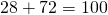we can figure out that the grown up is 28 years old.

Or, using algebra:# Two unknowns, two equations

Now suppose you ask my childrens’ ages. I have two children. I reply “I’m not telling you but if you add their ages together you get 10, and they are 4 years apart”.

The ages are still ‘unknown’ but because there are two different facts about the two ages, it is possible to figure them out.

To solve, we need to find two integers that add together to give 10, then find the one pair where the difference between them is four.  Here are all the options for adding to 10, written: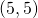The answer isasand.

To use algebra, we might say youngest child has ageand oldest has age. We have the following information:

Their ages add up to 10. That leads us to the equation, (or).

They are 4 years apart. That leads us to the equation, (or).

This unit is about how to find the values of two unknowns when we have two equations about them.

# Unique Solution

In the case about the two children, there was only one value ofandthat simultaneously solved the first and the second equation. This is called the unique solution. If we graph the two equations betweenandwe have:Notice that because the pointlies on both lines, it is the point of intersection of the two lines. Finding the solution to two equations in two unknowns boils down to finding the point where the two graphs intersect. If graphing is not efficient, an algebraic approach can be used.

# Identifying the unique Solution

Which ordered pairsatisfies both equations? Use mental arithmetic to determine which one of the three points lies on both lines.

# Infinitely Many Solutions

When two expressions are equivalent, all ordered pairs will satisfy both equations.

Considerand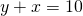The expression on the right is just a rearrangment of the expression on the left.

Using the relation, we can generate the following ordered pairs: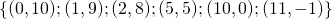…etc

Notice that all of those ordered pairs also satisfy.

As there are infinitely many points on any line, there are infinitely many ordered pairs that satisfy the systemIf one equation can be rearranged to the other, the equations are equivalent. All ordered pairs satisfying one equation will also satisfy the other.

# No Solutions

If our set of equations leads to two lines that are parallel but not coincident, then there is no ordered pair that will satisfy both relations.On a graph: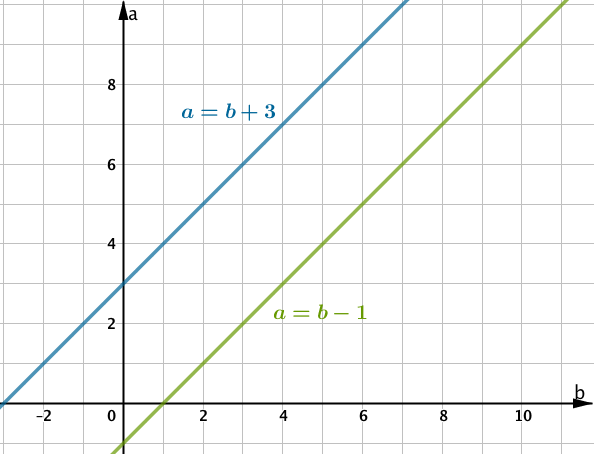Desmos Activities: Go to student.desmos.com, ask for the class code.

• Polygraph (requires pairs of students)
• Systems of Two Linear Equations (requires at least 4 students at a time)

Maths is Fun Page: